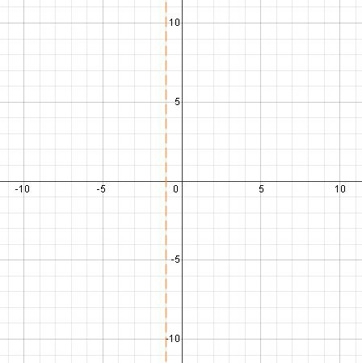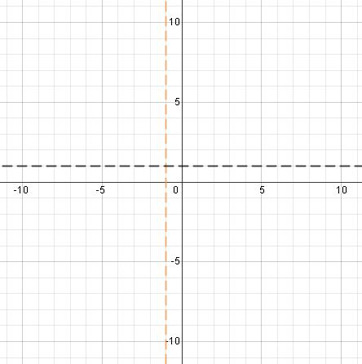# Graphing Rational Functions That Have Linear Polynomials: Steps & Examples

An error occurred trying to load this video.

Try refreshing the page, or contact customer support.

Coming up next: Graphing Rational Functions That Have Polynomials of Various Degrees: Steps & Examples

### You're on a roll. Keep up the good work!

Replay
Your next lesson will play in 10 seconds
• 0:01 Rational Functions…
• 1:19 Asymptotes
• 4:37 Points
• 5:40 The Graph
• 6:35 Lesson Summary

Want to watch this again later?

Timeline
Autoplay
Autoplay
Speed

#### Recommended Lessons and Courses for You

Lesson Transcript
Instructor: Yuanxin (Amy) Yang Alcocer

Amy has a master's degree in secondary education and has taught math at a public charter high school.

Watch this video lesson to learn how you can graph rational functions with linear polynomials in just a few steps. Also learn what kinds of functions these are and what you need to look for to graph them.

## Rational Functions with Linear Polynomials

The phrase 'rational functions with linear polynomials' is a mouthful, that's for sure. But don't worry, it's not as bad as it sounds. If we break the phrase down into its parts, we will see that it's not too bad. First, rational functions are simply fractions of polynomial functions. A linear polynomial is a polynomial whose highest exponent is 1. So a rational function with linear polynomials is a fraction of two polynomials whose highest degree is 1. Polynomials are those expressions that combine terms consisting of variables and their coefficients with pluses and minuses.

An example of a rational function with linear polynomial is the function f(x) = (x) / (x + 1). Look at the numerator and denominator, and we see that both are linear polynomials. Both the numerator and denominator consist of functions whose highest exponent is 1. A shortcut to identifying this type of function is just to look at the x. If both the numerator and denominator have an x with no exponent shown then you are looking at a rational function with linear polynomials. This is the type of function we will be graphing in this lesson.

## Asymptotes

The first step in graphing these functions is to look for asymptotes. We need to look for vertical asymptotes, the x values that make the function invalid, and horizontal asymptotes, the y value that the graph reaches for at the far left and far right of the graph.

To find our vertical asymptotes, we need to ask ourselves, what situation will make our function invalid? In other words, what situation causes the function to produce an error? Let's think about this. Our function is essentially a fraction. What do we know about fractions? What is the one number that we can never divide by? It's 0. So that tells us that if our denominator equals 0, then our function will produce an error.

So, at what x value does our denominator equal 0? How can we figure that out? We find this value by setting the denominator equal to 0. Our function is f(x) = (x) / (x + 1), and our denominator is x + 1. Setting this equal to 0, we get x + 1 = 0. Solve for this by subtracting 1 from both sides, we get x = -1. So our vertical asymptote is the vertical line at x = -1. We can draw this on our graph with a dashed line at x = -1.The next thing we look for is our horizontal asymptote. To find our horizontal asymptote, all we need to do is to look at our coefficients next to our variable x. In our case, the coefficients are 1 for the numerator and 1 for the denominator. So that means our horizontal asymptote is y = 1/1 = 1.

Yes, the horizontal asymptote is simply the fraction of the coefficients. How can you remember this? Think about the meaning of horizontal asymptote. It is the behavior of the graph at the far left and far right of the graph. This is where our x values are large. When our x is large, whatever we add or subtract won't make much difference, so we can ignore those numbers. Then since both the numerator and denominator have the same x, we can cancel them out. So what are we left with? We are left with the coefficients.

For our function f(x) = (x) / (x + 1), to find the horizontal asymptote, we ignored the + 1 on the bottom, which leaves us with y = x/x. We have the same x on the top and bottom, which tells us we can cancel them out. This leaves us with just the coefficients y = 1/1. We can draw our horizontal asymptote y = 1 using a dashed line as well.Now that we've drawn our asymptotes, we see that it separates our graph into four areas. One thing to note is that the graph will never touch or cross the vertical asymptote. So you will see the graph avoid this line like the plague! The horizontal asymptote, on the other hand, is like honey and attracts the graph. You will see the graph get closer and closer to this line.

To unlock this lesson you must be a Study.com Member.

### Register to view this lesson

Are you a student or a teacher?

### Unlock Your Education

#### See for yourself why 30 million people use Study.com

##### Become a Study.com member and start learning now.
Back
What teachers are saying about Study.com

### Earning College Credit

Did you know… We have over 160 college courses that prepare you to earn credit by exam that is accepted by over 1,500 colleges and universities. You can test out of the first two years of college and save thousands off your degree. Anyone can earn credit-by-exam regardless of age or education level.